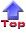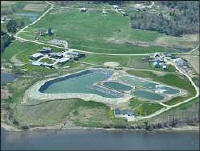Lagoon Systems In Maine

An Informational Resource for
Operators of Lagoon Systems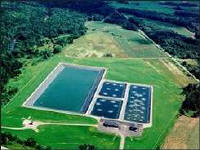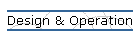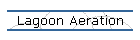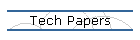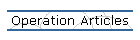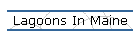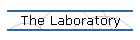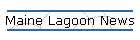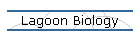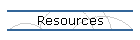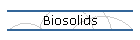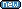2003 Maine Wastewater Salary Survey as conducted by the Maine Wastewater Control Association2003 Maine Wastewater Rate Survey conducted by the Maine Rural Water AssociationMaine DEP Monthly

Normality

By Tim Loftus

Normality, abbreviated as �N,� is a useful way of measuring the concentration of some solutions in the laboratory. While it is used in many areas of a laboratory technician�s world, we in the water and wastewater fields use it almost exclusively to measure the concentrations of acids and bases for such solutions as titrants in acidity and alkalinity analyses and for the pH adjustments of BOD, ammonia, and phosphorus samples.

Normality is similar in concept to molarity (refer to the previous article �Molarity�). Where molarity (M) represents the concentration of an ion or compound in solution, normality (N) goes one step further and represents the molar concentration only of the acid component (usually the H+ ion in an acid solution) or only the base component (usually the OH- ion in a base solution).

Here is a simple example to show the relationships of Normal acid and base solutions: a 1N solution of the acid H2SO4 will completely neutralize an equal volume of a 1N solution of the base NaOH. Even though the H2SO4 provides two (acid) H+ ions per molecule verses only one (base) OH- ion per NaOH molecule, the calculations of N take into account these differences and puts it all into an equivalent scale. In a sense, with normality calculations, you really can compare apples with oranges � acid and base-wise anyway.

If you know the Molarity of an acid or base solution, you can easily convert it to Normality by multiplying Molarity by the number of hydrogen (or hydroxide) ions in the acid (or base).

N = (M)(number of hydrogen or hydroxide ions)

For example, a 2 M H2SO4 solution will have a Normality of 4N (2 M x 2 hydrogen ions). A 2 M H3PO4, solution will have a Normality of 6N.

However, to make a solution of a predetermined normality requires a bit more calculating. First, you must determine the compound�s equivalent mass. This is done by taking the compound�s gram-molecular mass and dividing by the number of hydrogen ions or hydroxide ions. Here are a few examples:

H2SO4, sulfuric acid.
The gram-molecular mass is 98 (From the periodic chart the individual atomic masses are: H=1, S=32, O=16: {1x2}+32+{16x4}=98).
The number of acid hydrogen ions (H+ ) is 2.
Equivalent mass for H2SO4 is 98/2 = 49.

H3PO4, phosphoric acid. The gram-molecular mass is also 98. The number of hydrogen ions (H+ ) is 3. Equivalent mass for H3PO4 is 98/3 = 32.6.

NaOH, potassium hydroxide. The gram-molecular mass is 40. The number of hydroxide ions (OH-) is 1. Equivalent mass for NaOH is 40/1 = 40.

Once the equivalent mass of an acid or base is determined, you can then calculate the amount of grams needed per volume of water for N.

The formula to calculate this is:

Grams of compound needed = (N desired)(equivalent mass)(volume in liters desired).

For example, how many grams of sodium hydroxide would you need to dilute to a liter to make a 1N NaOH solution?

The equivalent mass is 40 as determined above.

Grams of NaOH needed = (1N)(40 eq. mass)(1 liter) = 40 grams of NaOH.

Similarly, to make 0.25 liters of a 0.05N potassium hydrogen phthalate (KHC8H4O4) solution (an acid), you would first determine the equivalent mass.

From the periodic chart, K=39, H=1, C=12, O=16. Its gram-molecular mass is 39+1+(12x8)+(1x4)+(16x4) = 204.

The number of hydrogen ions it can produce is 1 (The acid hydrogens are usually on the left side of a chemical formula. Hydrogens listed anywhere else usually don�t contribute to the �acid� part of the compound. In the case of KHC8H4O4, only the left most hydrogen is an �acid� hydrogen.) Its equivalent mass is 204/1 = 204.

To find the amount of potassium hydrogen phthalate (KHC8H4O4) needed to make 0.25 liters of a 0.05N solution:

Grams of KHC8H4O4 needed = (0.05N)(204 eq. mass)(0.25 liters) = 2.6 grams of KHC8H4O4.

Both of the chemicals in the examples above, sodium hydroxide and potassium hydrogen phthalate, are considered dry chemicals, which makes it relatively straightforward to calculate their Normalities. For liquid chemicals where the main compound is only a fraction of the total volume, such as the concentrated forms of hydrochloric (HCl), sulfuric (H2SO4), and phosphoric (H3PO4) acids, a few additional calculations must be performed to make a solution of a particular Normality.

The next article will describe and give examples of these additional calculations. These are not only useful for making acid and base solutions, but are useful in calculating concentrations of any type of concentrated dissolved compounds such as alum (aluminum sulfate), bleach (sodium hypochlorite), ferric chloride, and the many other solutions used in wastewater treatment.

Please note that this article specifically covers what is typically found in a wastewater treatment laboratory. There are exceptions to how the concentrations of acids and bases are measured, and this depends on the scope and application of a particular test method.

If you have any questions, suggestions, or comments, contact NEWEA Lab Practices Committee Chair Tim Loftus at (508) 949-3865 timloftus@msn.com. For more information on the NEWEA Laboratory Practices Committee, please contact Tim Loftus or Elizabeth Cutone, NEWEA Executive Director, 100 Tower Office Park, Woburn, MA 01801, (781) 939-0908, ecutone@newea.org.

All past articles are posted on our website. Go to www.NEWEA.org and follow the link to the Committee Pages then to the Laboratory Practices page.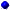Acidity and AlkalinityNormalityAmmonia NitrogenMaking Normal SolutionsBOD Test RequirementsPhosphorus 1BOD Test Requirements 2Phosphorus 2BOD Test Requirements 3QC SamplesChain of Custody ProcedureRecord KeepingChemical StorageRounding DigitsColiform and E. Coli Testing 1Sample ContaminationColiform and E. Coli Testing 2Significant DigitsColiform and E. Coli Testing 3Sample Handling for NPDESControl ChartsSigns of ToxicityDilution SolutionsSOPsMatters of Perspective pH  Part 1Total Chlorine ResidualMatters of Perspective pH  Part 2Total Chlorine Residual-2Method Detection LimitsTotal Suspended SolidsMolaritypH MeasurementsNitrogen